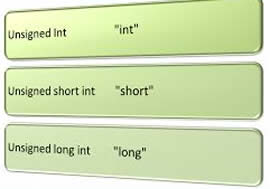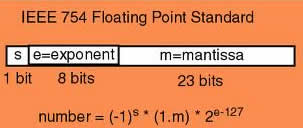# Difference between Integer and Float

##### Key Difference: Generally, Integers can be described as whole numbers meaning that they do not have any fractional parts, whereas float describes a number that can be only written in a decimal number system. In terms of data types, an integer belongs to a set of mathematical integers whose value is the same as a corresponding mathematical integer. Floats are floating point numbers that can be represented as a fixed number of significant digits and scaled using an exponent.Integer and float represent the values that are important building blocks in the field of arithmetic and computation. Integers refer to whole numbers. They do not have a fractional part. On the other hand, float defines the floating point values, which means that that they have decimal components in them. Integers and float are important data types used in various programming languages. The data used in programming has to be in some format. If the data is in the form of a whole number, it is assigned the tag of an integer.On the other hand, float represents the numbers that can be written in the scientific notation- with a base and an exponent. Float or floating point numbers possess a fixed specific number of bits which are arranged for the whole number and the fractional portion of the number. If the whole number portion of the number grows, then the bits for the fractions parts tend to be less available. All integers can be represented as floating point values. However, in programming due to the advantages like less memory occupancy, faster processing and rounding, integers are preferred. On the other hand, floats are used when single precision is required.

Comparison between Integer and Float:

 Integer Float Definition Integers can be described as whole numbers meaning that they do not have any fractional parts. Float or floating point numbers possess a fixed specific number of bits which are arranged for the whole number and the fractional portion of the number. Representation Integer data types can be represented in three number systems – Decimal, Octal and Hexadecimal Float can be only written using a decimal number system Memory requirement Generally, integer data types require 2 bytes of memory Generally, float data types require 4 bytes of memory Example -125, 567, 4,667 1.2e34, 0.98 Origin From Latin integer meaning literally "untouched," hence "whole" The location of decimal and binary point moves around, therefore named as floating numbers or float. Data type Integers Byte - 1 byte of information (8 bits), Range from -128 to 127 Short - 2 bytes, Range from -32768 to 32767 Int - 4 bytes, Range from -2147483648 to 2147483647 Long - 8 bytes, Range from -9,223,372,036,854,775,808  to 9,223,372,036,854,775,807 Occupies 32 bits (4 bytes) and its significand has a precision of 24 bits (about 7 decimal digits).

Image Courtesy: newtonapples.com, c-jump.com